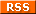Mascot
 Configuration The following configuration file is used in the examples: ```category code { open = true; useless_binding = true; string_literals = true; nested_for = { maximum = 2; } identifier_length = true; complex_condition = { maximum = 3; }; } category documentation { type_comment = true; value_comment = true; spell = true; } category interface { exported_count = { maximum = 2; }; } category metrics { coupling = true; } category miscellaneous { regexp = { expr = "Obj\\.magic"; case_sensitive = false; level = warning }; } category typography { tab_character = true; trailing_new_line = true; trailing_white_space = true; line_length = { maximum = 32; } } ``` Signature The following signature file is will produce these results: [ checkstyle ] [ html ] [ jdepend ] [ text ] [ xml ] ```(** Module descriptoion. *) type t (* This one is not actually documented (bare comment instead of ocamldoc one). *) val f : int -> int (** This one is documented. *) val g : int -> int val h : int -> int (** This one is also documented. *) ``` Structure The following structure file is will produce these results: [ checkstyle ] [ html ] [ jdepend ] [ text ] [ xml ] ```open Complex type t let f x = let a = [| |] in let len = List.length (Obj.magic a) in let len = len in len let g y = for i = 1 to y do for i = 1 to y do for i = 1 to y do print_endline "hello."; print_endline "hello."; print_endline "hello." done done done let h z = if (z > 1) || (z < 3) && (z >= 7) || (not (z = 2) || (z = 6)) then z * z else z + z ``` Both